Courses

# Test: Magnetostatics

## 10 Questions MCQ Test Topicwise Question Bank for Electronics Engineering | Test: Magnetostatics

Description
This mock test of Test: Magnetostatics for Electrical Engineering (EE) helps you for every Electrical Engineering (EE) entrance exam. This contains 10 Multiple Choice Questions for Electrical Engineering (EE) Test: Magnetostatics (mcq) to study with solutions a complete question bank. The solved questions answers in this Test: Magnetostatics quiz give you a good mix of easy questions and tough questions. Electrical Engineering (EE) students definitely take this Test: Magnetostatics exercise for a better result in the exam. You can find other Test: Magnetostatics extra questions, long questions & short questions for Electrical Engineering (EE) on EduRev as well by searching above.
QUESTION: 1

### The magnitude of H at a radius of 1 meter from along linear conductor is 1 A/m. The current in the wire is

Solution: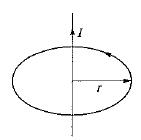Given, r = 1 m, H = 1 A/m
Using Ampere’s circuit law, we have: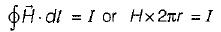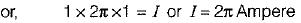QUESTION: 2

### What is the value of magnetic flux density at the centre of the square current loop shown in figure below?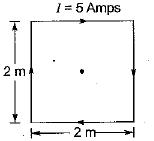Solution:

The magnetic flux density at the centre of the square having each side a is given by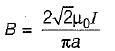Given, a = 2 m, I = 5 Amp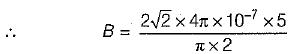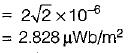QUESTION: 3

### A solenoid of 20 cm long and 1 cm diameter has a uniform winding of 1000 turns. If the solenoid is placed in a uniform field of 2 Wb/m2 flux density and a current of 10 amps, is passed through the solenoid winding, then the maximum torque on the solenoid will be

Solution:

Given, l = 20 cm = 0.2 m,
r - 0.5 cm = radius = 0.005 m
N = 1000,
B = 2 Wb/m2,
I = 10 A
The torque acting on the solenoid is
T = NIAB sinθ
T will be maximum when
θ = 90°, i.e. sin90° = 1
∴ Tmax = 1000 x 10 (0.005 x 0.2) x 2
= 20 N-m

QUESTION: 4

What is the value of magnetic flux density at the centre of a current carrying loop when the loop radius is 2 cm, loop current is 1 mA and the loop is placed in air?

Solution:

Magnetic flux density at the centre of a current carrying loop is given by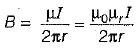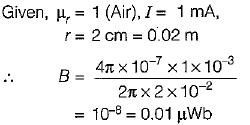QUESTION: 5

A magnetic field known to be directed in a cartesian co-ordinate system so that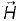exists in the x-direction where Hx = Constant. The value of curl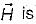Solution:

Curl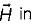cartesian coordinate is given by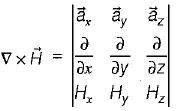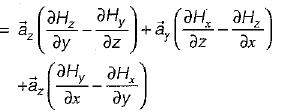Since Hx = constant and Hy = Hz = 0, therefore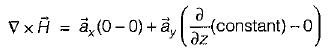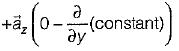= 0 + 0 + 0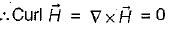QUESTION: 6

Match List-I with List-ll and select the correct answer using the codes given below the lists:
List-I
B. Maxwell equation in point form
C. Electric field strength general equation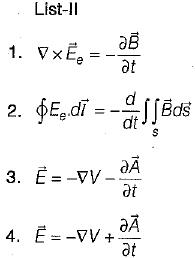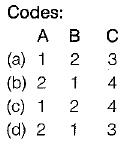Solution:
QUESTION: 7

The force acting between two parallel wires carrying currents I1 and I2 and separated by a distance r is given by

Solution:
QUESTION: 8

Flux lines are received at an iron-air boundary at an angle of 45° from the normal on the iron side of the boundary. If the iron has a relative permeability of 350, then the angle from the normal with which the flux emerges into the air would be

Solution: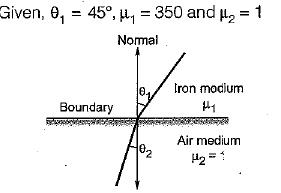Let medium-1 and medium-2 are respectively iron and air, then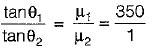or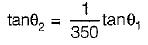or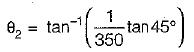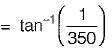QUESTION: 9

The unit of magnetic flux is given by

Solution:
QUESTION: 10

Assertion (A): The potential in case of magnetic field is a vector potential.
Reason (R): The potential in case of electric field is a scalar potential

Solution:
• Both assertion and reason are correct statements.
• The potential in case of magnetic field is a vector potential because source for producing a magnetic field is a current element which has both magnitude and direction.
• The potential in case of electric field i.e. electric potential is a scalar potential because the source for producing an electric field is a charge which is a scaler quantity (having only magnitude).